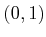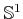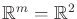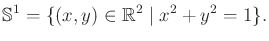#### 1D manifolds

The setof reals is the most obvious example of a 1D manifold becausecertainly looks like (via homeomorphism)in the vicinity of every point. The range can be restricted to the unit interval to yield the manifoldbecause they are homeomorphic (recall Example 4.5).

Another 1D manifold, which is not homeomorphic to, is a circle,. In this case, and let(4.5)

If you are thinking like a topologist, it should appear that this particular circle is not important because there are numerous ways to define manifolds that are homeomorphic to. For any manifold that is homeomorphic to, we will sometimes say that the manifold is, just represented in a different way. Also,will be called a circle, but this is meant only in the topological sense; it only needs to be homeomorphic to the circle that we learned about in high school geometry. Also, when referring to, we might instead substitutewithout any trouble. The alternative representations of a manifold can be considered as changing parameterizations, which are formally introduced in Section 8.3.2.

Steven M LaValle 2020-08-14